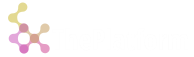Short-circuit conditional expression. Thus this verb is special. It does not evaluate all of its arguments immediately. Only the first argument is evaluated. If it is true, the second argument is evaluated and becomes the result of the `cond` expression. Otherwise, the third argument is evaluated.

Syntax: `\$[x;y;z]`

where `x` is a condition, `y` is an expression evaluated if `x` is true, `z` is an expression evaluated if `x` is false.

The concept of truth here is a bit complex:

• For boolean scalars, truth means 1b.
• For integer scalars, truth means any value except 0 and null.
• For other scalar types, truth means any non-null value.
• For vectors, dicts, tables, truth means non-empty structure.

``````o)\$[2<3;"yes";"no"]
"yes"
o)
o)\$[0n;"yes";"no"]
"no"
o)\$[();1;2]
2
o)t:([]a:1 2;b:1.1 2.2)
a b
-----
1 1.1
2 2.2
o)\$[t;1;0]
1
o)d:`a`b!(1 2 3;1.1 2.2 3.3)
a| 1 2 3
b| 1.1 2.2 3.3
o)\$[d;1;0]
1
o)
``````

Another thing to remember is that simulation of short circuit evaluation of condition itself is done using nested conds:

``````o) a:1 2 3;
o) \$[a;\$[1=a;2;3];4]
2
o)t:([]a:1 2;b:1.1 2.2)
a b
-----
1 1.1
2 2.2
o)\$[3=count t;\$[d[`b;1]=2.2;1;2];\$[d[`a;0]=0;3;4]]
3
o)
``````